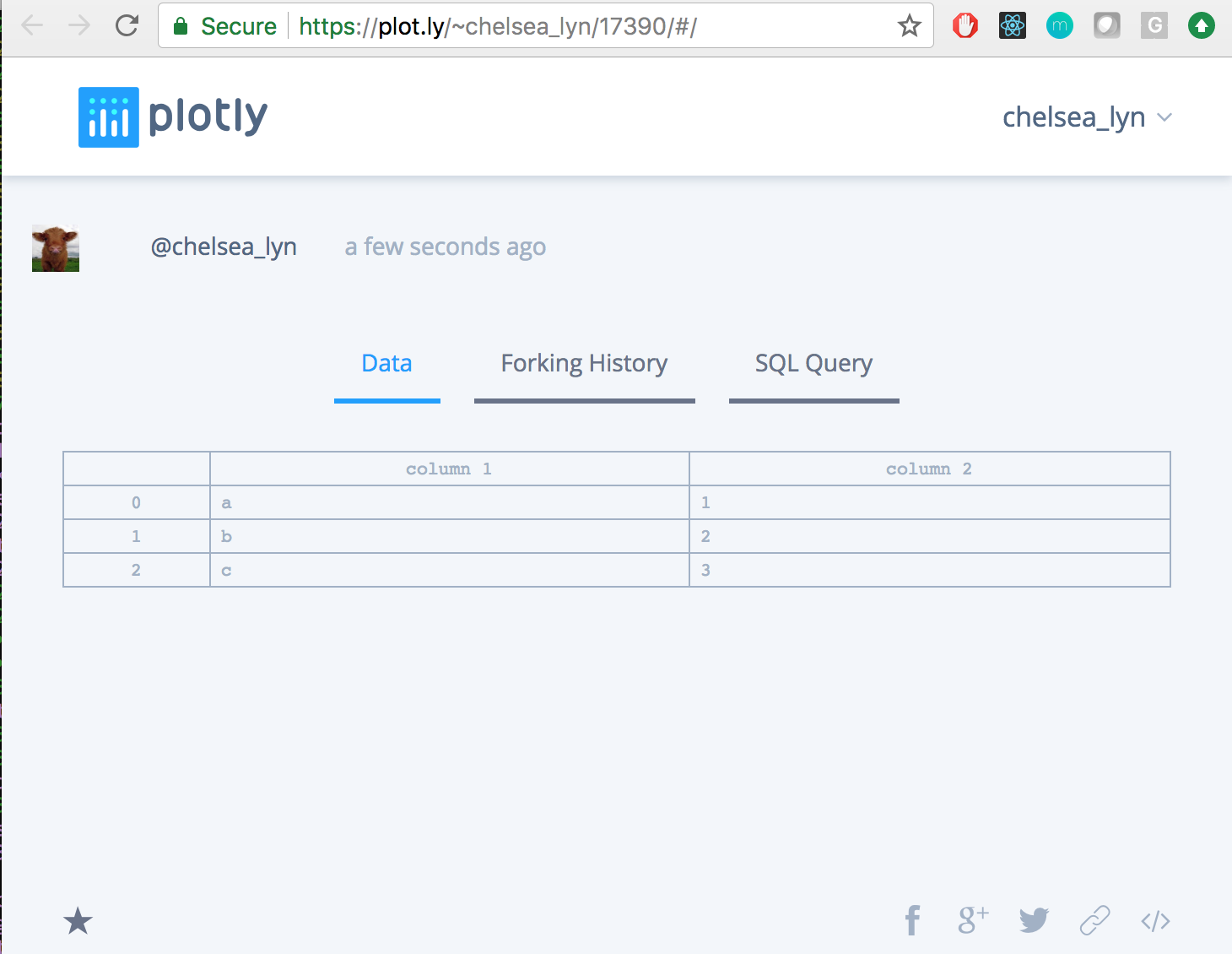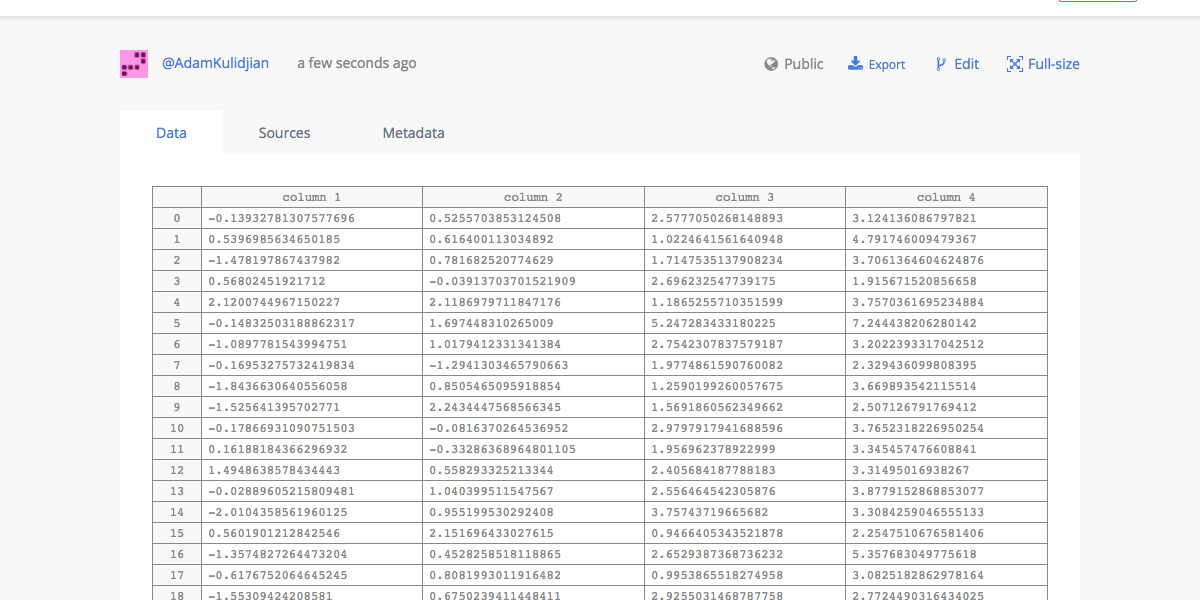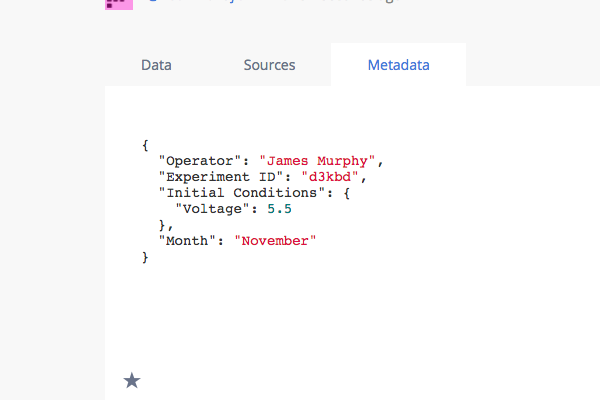# Plots from Grids in Python/v3

How to upload data to Plotly from Python with the Plotly Grid API.

See our Version 4 Migration Guide for information about how to upgrade.

#### New to Plotly?Â¶

You can set up Plotly to work in online or offline mode, or in jupyter notebooks.
We also have a quick-reference cheatsheet (new!) to help you get started!

#### Creating a Plotly GridÂ¶

You can instantiate a grid with data by either uploading tabular data to Plotly or by creating a Plotly grid using the API. To upload the grid we will use plotly.plotly.grid_ops.upload(). It takes the following arguments:

• grid (Grid Object): the actual grid object that you are uploading.
• filename (str): name of the grid in your plotly account,
• world_readable (bool): if True, the grid is public and can be viewed by anyone in your files. If False, it is private and can only be viewed by you.
• auto_open (bool): if determines if the grid is opened in the browser or not.

You can run help(py.grid_ops.upload) for a more detailed description of these and all the arguments.

In :
import plotly
import plotly.plotly as py
import plotly.tools as tls
import plotly.graph_objs as go
from plotly.grid_objs import Column, Grid

from datetime import datetime as dt
import numpy as np
from IPython.display import Image

column_1 = Column(['a', 'b', 'c'], 'column 1')
column_2 = Column([1, 2, 3], 'column 2') # Tabular data can be numbers, strings, or dates
grid = Grid([column_1, column_2])
filename='grid_ex_'+str(dt.now()),
auto_open=False)
print(url)

https://plotly.com/~chelsea_lyn/17398/


#### View and Share your GridÂ¶

You can view your newly created grid at the url:

In :
Image('view_grid_url.png')

Out:You are also able to view the grid in your list of files inside your organize folder.

Along with uploading a grid, you can upload a Dataframe as well as convert it to raw data as a grid:

In :
import plotly.plotly as py
import plotly.figure_factory as ff

import pandas as pd

py.iplot(table, filename='dataframe_ex_preview')

Out:
In :
grid = Grid([Column(df[column_name], column_name) for column_name in df.columns])
print(url)

https://plotly.com/~chelsea_lyn/17399/


#### Making Graphs from GridsÂ¶

Plotly graphs are usually described with data embedded in them. For example, here we place x and y data directly into our Histogram2dContour object:

In :
x = np.random.randn(1000)
y = np.random.randn(1000) + 1

data = [
go.Histogram2dContour(
x=x,
y=y
)
]

py.iplot(data, filename='Example 2D Histogram Contour')

Out:

We can also create graphs based off of references to columns of grids. Here, we'll upload several columns to our Plotly account:

In :
column_1 = Column(np.random.randn(1000), 'column 1')
column_2 = Column(np.random.randn(1000)+1, 'column 2')
column_3 = Column(np.random.randn(1000)+2, 'column 3')
column_4 = Column(np.random.randn(1000)+3, 'column 4')

grid = Grid([column_1, column_2, column_3, column_4])
print(url)

https://plotly.com/~chelsea_lyn/17400/

In :
Image('rand_int_histogram_view.png')

Out:#### Make Graph from Raw DataÂ¶

Instead of placing data into x and y, we'll place our Grid columns into xsrc and ysrc:

In :
data = [
go.Histogram2dContour(
xsrc=grid,
ysrc=grid
)
]

py.iplot(data, filename='2D Contour from Grid Data')

Out:

So, when you view the data, you'll see your original grid, not just the columns that compose this graph:

#### Attaching Meta Data to GridsÂ¶

In Chart Studio Enterprise, you can upload and assign free-form JSON metadata to any grid object. This means that you can keep all of your raw data in one place, under one grid.

If you update the original data source, in the workspace or with our API, all of the graphs that are sourced from it will be updated as well. You can make multiple graphs from a single Grid and you can make a graph from multiple grids. You can also add rows and columns to existing grids programatically.

In :
meta = {
"Month": "November",
"Experiment ID": "d3kbd",
"Operator": "James Murphy",
"Initial Conditions": {
"Voltage": 5.5
}
}

print(url)

https://plotly.com/~chelsea_lyn/17400/

In :
Image('metadata_view.png')

Out:#### ReferenceÂ¶

In :
help(py.grid_ops)

Help on class grid_ops in module plotly.plotly.plotly:

class grid_ops
|  Interface to Plotly's Grid API.
|  Plotly Grids are Plotly's tabular data object, rendered
|  references of columns of Plotly grid objects. Free-form
|  JSON Metadata can be saved with Plotly grids.
|
|  To create a Plotly grid in your Plotly account from Python,
|  see grid_ops.upload.
|
|  To add rows or columns to an existing Plotly grid, see
|  grid_ops.append_rows and grid_ops.append_columns
|  respectively.
|
|  To delete one of your grid objects, see grid_ops.delete.
|
|  Class methods defined here:
|
|  append_columns(cls, columns, grid=None, grid_url=None) from __builtin__.classobj
|      Append columns to a Plotly grid.
|
|      columns is an iterable of plotly.grid_objs.Column objects
|      and only one of grid and grid_url needs to specified.
|
|      grid is a ploty.grid_objs.Grid object that has already been
|
|      grid_url is a unique URL of a grid in your plotly account.
|
|      Usage example 1: Upload a grid to Plotly, and then append a column
|      
|      from plotly.grid_objs import Grid, Column
|      import plotly.plotly as py
|      column_1 = Column([1, 2, 3], 'time')
|      grid = Grid([column_1])
|
|      # append a column to the grid
|      column_2 = Column([4, 2, 5], 'voltage')
|      py.grid_ops.append_columns([column_2], grid=grid)
|      
|
|      Usage example 2: Append a column to a grid that already exists on
|                       Plotly
|      
|      from plotly.grid_objs import Grid, Column
|      import plotly.plotly as py
|
|      grid_url = 'https://plotly.com/~chris/3143'
|      column_1 = Column([1, 2, 3], 'time')
|      py.grid_ops.append_columns([column_1], grid_url=grid_url)
|      
|
|  append_rows(cls, rows, grid=None, grid_url=None) from __builtin__.classobj
|      Append rows to a Plotly grid.
|
|      rows is an iterable of rows, where each row is a
|      list of numbers, strings, or dates. The number of items
|      in each row must be equal to the number of columns
|      in the grid. If appending rows to a grid with columns of
|      unequal length, Plotly will fill the columns with shorter
|      length with empty strings.
|
|      Only one of grid and grid_url needs to specified.
|
|      grid is a ploty.grid_objs.Grid object that has already been
|
|      grid_url is a unique URL of a grid in your plotly account.
|
|      Usage example 1: Upload a grid to Plotly, and then append rows
|      
|      from plotly.grid_objs import Grid, Column
|      import plotly.plotly as py
|      column_1 = Column([1, 2, 3], 'time')
|      column_2 = Column([5, 2, 7], 'voltage')
|      grid = Grid([column_1, column_2])
|
|      # append a row to the grid
|      row = [1, 5]
|      py.grid_ops.append_rows([row], grid=grid)
|      
|
|      Usage example 2: Append a row to a grid that already exists on Plotly
|      
|      from plotly.grid_objs import Grid
|      import plotly.plotly as py
|
|      grid_url = 'https://plotly.com/~chris/3143'
|
|      row = [1, 5]
|      py.grid_ops.append_rows([row], grid=grid_url)
|      
|
|  delete(cls, grid=None, grid_url=None) from __builtin__.classobj
|      Delete a grid from your Plotly account.
|
|      Only one of grid or grid_url needs to be specified.
|
|      grid is a plotly.grid_objs.Grid object that has already
|
|      grid_url is the URL of the Plotly grid to delete
|
|      Usage example 1: Upload a grid to plotly, then delete it
|      
|      from plotly.grid_objs import Grid, Column
|      import plotly.plotly as py
|      column_1 = Column([1, 2, 3], 'time')
|      column_2 = Column([4, 2, 5], 'voltage')
|      grid = Grid([column_1, column_2])
|
|      # now delete it, and free up that filename
|      py.grid_ops.delete(grid)
|      
|
|      Usage example 2: Delete a plotly grid by url
|      
|      import plotly.plotly as py
|
|      grid_url = 'https://plotly.com/~chris/3'
|      py.grid_ops.delete(grid_url=grid_url)
|      
|
|      Upload a grid to your Plotly account with the specified filename.
|
|      Positional arguments:
|          - grid: A plotly.grid_objs.Grid object,
|                  call help(plotly.grid_ops.Grid) for more info.
|          - filename: Name of the grid to be saved in your Plotly account.
|                      To save a grid in a folder in your Plotly account,
|                      separate specify a filename with folders and filename
|                      separated by backslashes (/).
|                      If a grid, plot, or folder already exists with the same
|                      filename, a plotly.exceptions.RequestError will be
|                      thrown with status_code 409
|
|      Optional keyword arguments:
|          - world_readable (default=True): make this grid publically (True)
|                                           or privately (False) viewable.
|          - auto_open (default=True): Automatically open this grid in
|                                      the browser (True)
|          - meta (default=None): Optional Metadata to associate with
|                                 this grid.
|                                 JSON-encodable object, for example:
|                                 {"experiment name": "GaAs"}
|
|      Filenames must be unique. To overwrite a grid with the same filename,
|      you'll first have to delete the grid with the blocking name. See
|      plotly.plotly.grid_ops.delete.
|
|      Usage example 1: Upload a plotly grid
|      
|      from plotly.grid_objs import Grid, Column
|      import plotly.plotly as py
|      column_1 = Column([1, 2, 3], 'time')
|      column_2 = Column([4, 2, 5], 'voltage')
|      grid = Grid([column_1, column_2])
|      
|
|      Usage example 2: Make a graph based with data that is sourced
|                       from a newly uploaded Plotly grid
|      
|      import plotly.plotly as py
|      from plotly.grid_objs import Grid, Column
|      from plotly.graph_objs import Scatter
|      column_1 = Column([1, 2, 3], 'time')
|      column_2 = Column([4, 2, 5], 'voltage')
|      grid = Grid([column_1, column_2])
|
|      # Build a Plotly graph object sourced from the
|      # grid's columns
|      trace = Scatter(xsrc=grid, ysrc=grid)
|      py.plot([trace], filename='graph from grid')
|      
|
|  ----------------------------------------------------------------------
|  Static methods defined here:
|